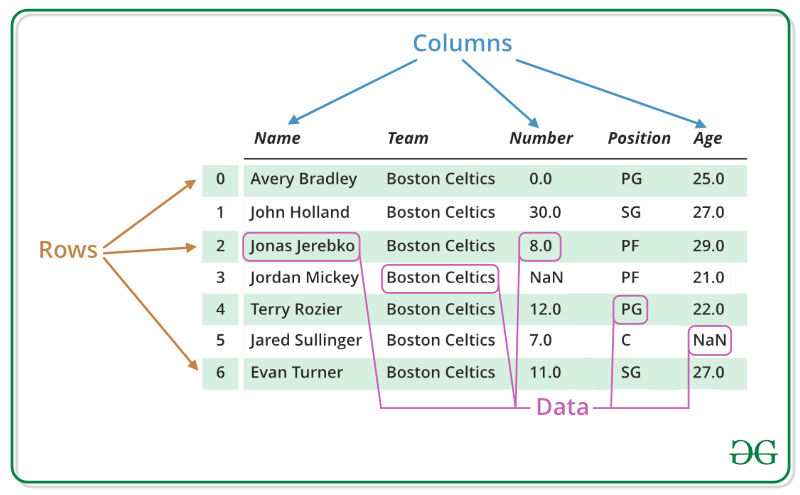# An Introduction to the Pandas Library

By George Bennett

Pandas is a library in python that helps with organizing and exploring data. It is built on the numpy library. Pandas stores data in neat tables called “DataFrames” that can be easily manipulated. I will be explaining how to create dataframes, how to quickly get information from dataframes with slicing, how to make quick plots, and how to export data into a .csv file.taken from https://media.geeksforgeeks.org/wp-content/uploads/finallpandas.png

To create a dataframe out of a .csv file simply call the pandas .read_csv method with the file name passed in to save it as a variable. This is the most common way to import data into a dataframe. You can view the entire dataframe by calling the variable, or you can view just the top 5 lines by calling .head on the dataframe.

`import pandas as pddf = pd.read_csv("my_data.csv")df.head()`

If you want to create a dataframe for simple display purposes pass a dictionary into the pandas method .DataFrame. Each key in the dictionary is the name of a column and the lists should be correctly ordered as they are they fill in all the data for the column.

You can quickly run conditional statements on the data or “slice” it to get the data you need to answer a question. Slice a column out by putting brackets after the dataframe and put the name of that column as a string, or a list of names of columns inside the brackets. This will return those columns. For conditionals pass the dataframe followed by brackets with a conditional inside the brackets. For instance if you needed a slice of the dataframe that only contained rows with a “price” value over \$5.00 you could save that to a variable as a new smaller dataframe.

`prices_over_5 = dataframe[dataframe["price"] > 5]`

To slice the dataframe by index you can use .loc and .iloc. If you only need certain columns call .loc with brackets and pass in the indexes needed, followed by a comma, followed by the columns needed . You can put the indexes in the form of a list or a range. .iloc is like .loc except it only takes in index values.

`first_five_col1_col2 = df.loc[:5, ["column1", "column2"]`

Pandas has matplotlib built into it for plotting graphs. One great graph to use is the scatter matrix. This graph plots each column against each other in a scatter plot form. The scatter plots form a matrix and the diagonal shows a histogram of each column. This allows one to quickly check for linear relationships and see the distributions of each column. To make this plot call the pandas alias followed by .plotting, followed by the .scatter_matrix method. You can optionally pass in a list of columns in order to only include those columns. You can also change the amount of space the plot occupies.

To save a dataframe back into a .csv file call .to_csv on the dataframe and pass in the file name. Make sure to set the index parameter to false.

`df.to_csv("my_data.csv", index=False)`

More pandas operations will be covered in my next post so stay tuned!

Data scientist learning at Flat Iron School

## More from Datascience George

Data scientist learning at Flat Iron School